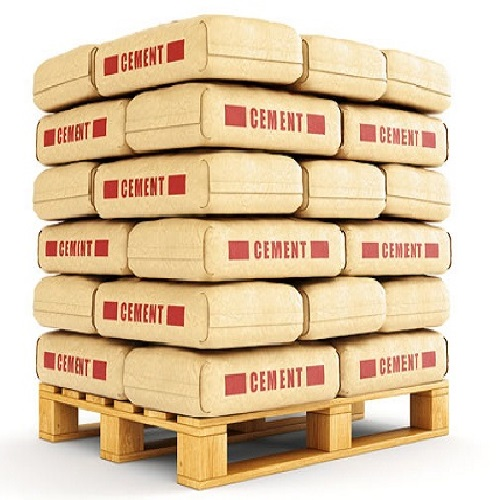#### Online Construction Cost Calculator :Online Construction Cost
Builtup Area (Sq.ft) Approx Cost (Per Sq.ft) Approx amount of construction is :Cement Required :Cement Amount :Sand Required :Sand Amount :Aggregate Required :Aggregate Amount :Steel Required :Steel Amount :Paint Required :Bricks Required :Floorings : Finishers : Fittings :
##### Construction Cost :
• Construction Cost calculator is used to calculate the Approx amount of construction cost and total cost.
• In addition to the number of Total Amount, Cement Required and its Amount, Sand Required and its Amount, Aggregate Required and its Amount, Steel Required and its Amount, Paint, Brick Required, Flooring, Finishers, Fittings are also will be calculated.
• Likewise, The amount of the cement and sand and aggregate and steel will be change daily.
• So, The amount will be in approximate rate.
• Then, the online construction cost calculator is one of the civil calculator.
##### Description :
• The construction area is also known as a built-up area.
• Therefore, Constructing a house is a huge task.
• Then, you have to be on your toes with almost everything that is happening around, the cost, raw materials, labour, resources and every minute detail.
• When you are handed over the task to oversee everything, it is most likely that you will start with the cost factor.
• The construction area consists of all carpet areas, plus common areas such as staircases, lobbies service areas etc.
• To calculate the construction area one would either need a plan or else an estimate from previous development or experience.
• Moreover the cost of construction is often measured and evaluated by its cost per square foot, you may want to determine this number for your construction project.
• Once your total construction cost is determined, calculating the cost per square foot is as simple as dividing the cost by the total square footage of the project.
##### Construction Cost Calculation Formula :
• To find the cost.
• Approx amount of construction cost = Builtup area * Approx cost.
• Cement Required = Builtup area * 0.4.
• Then, Cement Amount = (16.4/100) * Approx amount of cost.
• Sand Required = Builtup Area * 0.816.
• Then, Sand Amount = (12.3/100) * Approx amount of cost.
• Aggregate Required = Builtup Area * 0.608.
• Then, Aggregate Amount = (7.4/100) * Approx amount of cost.
• Steel Required = Builtup Area * 4.
• Then, Steel Amount = (24.6/100) * Approx. amount of cost.
• Paint Required = Builtup Area * 0.18.
• Brick Required = Builtup Area * 8.
• Floorings = Builtup Area * 1.3.
• Finishers = (16.5 / 100) * Approx amount of cost.
• Fittings = (22.8 / 100) *Approx amount of cost.
• Lastly, the total amount will be calculated and shown at last.
• Besides, the formula this calculator works.
##### How to Calculate ?
• First, Enter the Builtup Area of sq.ft.
• Second, Enter the Approx Cost per sq.ft.
• Click “Calculate” button then you will get the result and In addition to clear values Click “Reset”.
• Finally, follow these steps to get the results.
##### Other Calculators :
Get it or Get it done!

Get a free Revit family by requesting it. For the first 100 persons* the next day.

*Conditions apply​

Hi Myself Raj,
Do you need any BIM Modelling Services